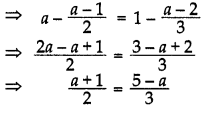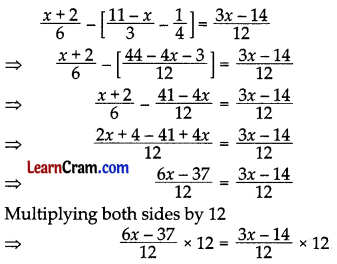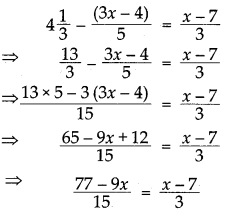# DAV Class 7 Maths Chapter 7 Brain Teasers Solutions

The DAV Maths Book Class 7 Solutions Pdf and DAV Class 7 Maths Chapter 7 Brain Teasers Solutions of Linear Equations in One Variable offer comprehensive answers to textbook questions.

## DAV Class 7 Maths Ch 7 Brain Teasers Solutions

Question 1.
A. Tick (✓) the correct option:
(i) x + x + x + x = _____________.
(a) x4
(b) 4x
(c) x
(d) 4x4
(b) 4x

x + x + x + x = 4x
Hence, (b) is the correct answer.

(ii) (9z + 7) – 5(2z – 3) + 3(2z + 3) = _____________
(a) 5z + 31
(b) -5z + 31
(c) 5z-31
(d) -5z-31
(9z + 7) – 5 (2z – 3) + 3(2z + 3)
= 9z + 7 – 10z + 15 + 6z + 9
= 5z + 31
Hence, (a) is the correct answer.

(iii) If 4x – 3 = 21, what is the value of(3x – 5)?
(a) 16
(b) 14
(c) 13
(d) 15
4x – 3 = 21 (given)
4x = 21 + 3
⇒ 4x = 24
⇒ x = $$\frac{24}{4}$$
x = 6
Now, putting x = 6 in (3x – 5), we have 3(6) – 5 = 18 – 5 = 13
Hence, (c) is the correct answer.

(iv) Which of the following is a solution of the equation —
3x – 7 = 7 – 4x
(a) x = 0
(b) x = 14
(c) x = 2
(d) x = 1
3x – 7 = 7- 4x
3x + 4x = 7 + 7
7x = 14
x = $$\frac{14}{7}$$
x = 2
Hence, (c) is the correct option.

(v) x = 5 is a solution of the equation —
(a) $$\frac{3}{5}$$x – 6 = 3
(b) 2x + 2 = 4x
(c) 4x – 2 = 3x + 3
(d) 3(x – 3) = 5(2x + 1)
Putting x = 5 in 4x – 2 = 3x + 3, we have
4(5) – 2 = 3(5) + 3
⇒ 20 – 2 = 15 + 3
⇒ 18 = 18, which is true.
So, x = 5 satisfies the equation 4x – 2 = 3x + 8.
Hence, (c) is the correct answer.(i) A confectioner had some boxes for burger and he bought 50 more boxes. After two days half of these boxes were used and only 40 boxes were left. Find the number of boxes he had in the beginning.
Let the number of boxes confectioner had in the beginning be x.
Now, according to the question, we have
(x + 50) – $$\frac{1}{2}$$(x + 50) = 40
⇒ $$\frac{1}{2}$$(x + 50) = 40
⇒ x + 50 = 80
⇒ x = 80 – 50 = 30
Therefore, the confectioner had 30 boxes in the beginning.

(ii) Find K, so that x = 3 is a solution of the equation Kx + 8 = x – 7.
x = 3 is a solution of Kx + 8 = x- 7
⇒ K(3) + 8=3-7
⇒ 3K + 8 = -4
⇒ 3K = -4 – 8
⇒ 3K = -12
⇒ K = $$\frac{-12}{3}$$
⇒ K = -4

(iii) Seema had a wire of some length. After making a square of side 4 cm with it, 5 cm wire left. Find the length of wire Seema had.
Let the length of wire Seema had be x cm.
Now, according to the question, we have
x – 4(4) = 5
x – 16 = 5
x = 16 + 5
x = 21
Therefore, Seema had wire of 21 cm length.

(iv) If A = (2a – 3) and B = (5 – 3a) be two algebraic expressions such that 2 A + B = 6, then find the value of a.
Given : A = (2a – 3)
and B = (5 – 3a)
Now, 2A + B = 6 …(1)
On putting the values of A = (2a – 3) and B = (5 – 3a) in equation(1), we have
2(2a – 3) + (5 – 3a) = 6
⇒ 4a – 6 + 5 – 3a = 6
⇒ a – 1 = 6
⇒ a = 6 + 1
⇒ a = 7

(v) Check whether y = l is a solution of the equation —
1.2(3y – 4) – 0.3(2y – 6) = 0 or not.
On putting y = 1 in LHS of the given equation, we have
LHS = 1.2[3(1) – 4] – 0.3[2(1) – 6]
= 1.2(3 – 4) – 0.3(2 – 6)
= 1.2(-1) – 0.3(-4)
= -1.2 + 1.2 = 0
= RHS
As LHS = RHS, so y = 1 is a solution of the given equation.

Question 2.
Solve the following equations:
(i) $$\frac{2 x+14}{3 x+6}$$ = 7
⇒ $$\frac{6 x+4 x-3 x}{12}$$ = 7
⇒ $$\frac{7 x}{12}$$ = 7
⇒ x = 7 × $$\frac{12}{7}$$
⇒ x = 12
Hence, x = 12

(ii) $$\frac{2}{3}$$(y – 5) – $$\frac{1}{4}$$(y – 2) = $$\frac{9}{2}$$(iii) 13(z – 4) – 3 (z – 9) – 5(z + 4) = 0
13(z – 4) – 3 (z – 9) – 5 (z + 4) = 0
⇒ 13z – 52 – 3z + 27 – 5z – 20 = 0
⇒ 13z – 3z – 5z = 52 – 27 + 20
⇒ 5z = 45
⇒ z = $$\frac{45}{5}$$ = 9
Hence z = 9

(iv) (x + 2)(x + 3) + (x – 3)(x – 2) – 2x(x + 1) = 0
(x + 2)(x + 3) + (x – 3)(x – 2) – 2x(x + 1) = 0
⇒ x(x + 3) + 2(x + 3) + x(x – 2) – 3(x – 2) – 2x(x + 1) = 0
⇒ x2 + 3x + 2x + 6 + x2 – 2x – 3x + 6 – 2x2 – 2x = 0⇒ -2x = -12
⇒ x = $$\frac{-12}{-2}$$ = 6
Hence x = 6.

(v) $$\frac{2 x+14}{3 x+6}$$ = 4
⇒ 2x + 14 = 4(3x + 6)
⇒ 2x + 14 = 12x + 24
⇒ 2x – 12x = 24-14
⇒ – 10x = -10
⇒ x = $$\frac{-10}{2}$$ = 1
Hence x = 1

(vi) a – $$\left(\frac{a-1}{2}\right)$$ = 1 – $$\left(\frac{a-2}{3}\right)$$⇒ 3(a + 1) = 2(5 -a )
⇒ 3a + 3 = 10 – 2a
⇒ 3a + 2a = 10 – 3
⇒ 5a = 7
⇒ a = $$\frac{7}{5}$$
Hence a = $$\frac{7}{5}$$

(vii) $$\frac{x+2}{6}-\left[\frac{11-x}{3}-\frac{1}{4}\right]=\frac{3 x-14}{12}$$⇒ 6x – 37 = 3x – 14
⇒ 6x – 3x = 37 -14
⇒ 3x = 23
⇒ x = 23
⇒ x = $$\frac{23}{3}$$
Hence x = $$\frac{23}{3}$$

(viii) $$\frac{2}{3 x}$$ – 1 = $$\frac{1}{12}$$
⇒ $$\frac{2}{3 x}$$ = 1 + $$\frac{1}{12}$$
⇒ $$\frac{2}{3 x}=\frac{12+1}{12}$$
⇒ $$\frac{2}{3 x}=\frac{13}{12}$$
⇒ 3x x 13 = 2 x 12
⇒ 39x = 24
⇒ x = $$\frac{24}{39}=\frac{8}{13}$$
Hence z = 9.Question 3.
The numerator of a fraction is 6 less than the denominator. If 3 is added to the numerator, the fraction becomes equal to $$\frac{2}{3}$$. Find the original fraction.
Let the denominator be x
∴ Numerator = x – 6
As per the condition = $$\frac{x-6+3}{x}=\frac{2}{3}$$
⇒ $$\frac{x-3}{x}=\frac{2}{3}$$
⇒ 3x – 9 = 2x
⇒ 3x – 2x = 9
⇒ x = 9
Denominator = 9
and Numerator = 9 – 6 = 3
Hence the original fraction = $$\frac{3}{9}$$

Question 4.
A man travelled y th of his journey by train, $$\frac{1}{3}$$rd by taxi, $$\frac{1}{6}$$ th by bus and the remaining 10 km on foot. Find the length of his total journey.
Let the total journey be x km
Distance travelled by train = $$\frac{2}{5}$$x km
Distance travelled by taxi = $$\frac{1}{3}$$x km
Distance travelled by bus = $$\frac{1}{6}$$x km
Distance travelled on foot = 10 km
x = $$\frac{2}{5}$$x + $$\frac{1}{3}$$x + $$\frac{1}{6}$$x + 10
⇒ x – $$\frac{2}{5}$$x – $$\frac{1}{3}$$x – $$\frac{1}{6}$$x = 10
⇒ $$\frac{30 x-12 x-10 x-5 x}{30}$$ = 10
⇒ 30x – 27x = 10 × 30
⇒ 3x = 300
⇒ x = = 100
Hence the length of total journey = 100 km

Question 5.
A table costs ₹ 200 more than a chair. The price of 2 tables and 3 chairs is ₹ 1400. Find the cost of each.
Let the cost of chair be ₹ x
Cost of table = ₹ (x + 200)
2(x + 200) + 3x = 1400
⇒ 2x + 400 + 3x = 1400
⇒ 5x = 1400 – 400
⇒ 5x = 1000
⇒ x = $$\frac{1000}{5}$$ = 200
Hence the cost of the chair = ₹ 200
and the cost of the table = ₹ 200 + ₹ 200 = ₹ 400.

Question 6.
Anu is 4 years older than Sunil. Eight years ago, Anu was three times Sunil’s age. Find the ages of Anu and Sunil.
Let the age of Sunil be x years
∴ Age of Anu = (x + 4) years.
8 years ago, Sunil’s age = (x – 8) years
8 years ago, Anu’s age = (x + 4 – 8) years
= (x – 4) years
As per condition, x – 4 = 3 (x – 8)
⇒ x – 4 = 3x – 24
⇒ x – 3x = – 24 + 4
⇒ -2x = -20
⇒ x = $$\frac{-20}{-2}$$ = 10
Hence Sunil’s age = 10 years,
and Anu’s age = 10 + 4 = 14 years.

Question 1.
Solve : (x – 4) (x + 4) = (x + 3) (x – 7) + 35
(x – 4) (x + 4) = (x + 3) (x – 7) + 35
⇒ x(x + 4) – 4 (x + 4) = x(x – 7) + 3 (x – 7) + 35⇒ -16 = -4x + 14
⇒ 4x = 16 + 14
⇒ 4x = 30
⇒ x = $$\frac{30}{4}=\frac{15}{2}$$
Hence solution is x = $$\frac{15}{2}$$

Question 2.
Solve: $$\frac{2}{3}$$(x – 3) = 1 – $$\frac{5}{6}$$(3x – 4)
$$\frac{2}{3}$$(x – 3) = 1 – $$\frac{5}{6}$$(3x – 4)
⇒ $$\frac{2}{3}$$(x – 3) + $$\frac{5}{6}$$(3x – 4) = 1
⇒ $$\frac{4(x-3)+5(3 x-4)}{6}$$ = 1
⇒ 4x – 12 + 15x – 20 = 6
⇒ 19x – 32 = 6
⇒ 19x = 6 + 32
⇒ 19x = 38
⇒ x = $$\frac{38}{19}$$ = 2
Hence solution is x = 2

Question 3.
Solve: 4$$\frac{1}{3}$$ – $$\frac{(3 x-4)}{5}=\frac{x-7}{3}$$⇒ 3(77 – 9x) = 15(x – 7)
⇒ 231 – 27x = 15x – 105
⇒ – 27x – 15x = – 105 – 231
⇒ – 42x = -336 – 336
⇒ x = $$\frac{-336}{-42}$$ = 8
Hence solution is x = 8

Question 4.
Solve: $$\frac{7}{x-4}=\frac{5}{x+2}$$
$$\frac{7}{x-4}=\frac{5}{x+2}$$
⇒ 7(x + 2) = 5(x – 4)
⇒ 7x + 14 = 5x – 20
⇒ 7x – 5x = -14 – 20
⇒ 2x = – 34
⇒ x = $$\frac{-34}{2}$$ = -17
Hence x = -17Question 5.
Solve: $$\frac{5 x-7}{4}-\frac{2 x-5}{3}=\frac{5 x}{6}$$
⇒ $$\frac{3(5 x-7)-4(2 x-5)}{12}=\frac{5 x}{6}$$
⇒ $$\frac{15 x-21-8 x+20}{12}=\frac{5 x}{6}$$
⇒ $$\frac{7 x-1}{12}=\frac{5 x}{6}$$
6(7x – 1) = 12 x 5x
42x – 6 = 60x
42x – 60x = 6
-18x = 6
x = $$\frac{-6}{18}=\frac{-1}{3}$$
Hence x = $$\frac{-1}{3}$$

Question 6.
Solve: $$\frac{2 x+1}{10}-\frac{3-2 x}{15}=\frac{x-2}{6}$$
⇒ $$\frac{3(2 x+1)-2(3-2 x)}{30}=\frac{x-2}{6}$$
⇒ $$\frac{6 x+3-6+4 x}{30}=\frac{x-2}{6}$$
⇒ $$\frac{10 x-3}{30}=\frac{x-2}{6}$$
⇒ 6(10x – 3) = 30(x – 2)
⇒ 60x – 18 = 30x – 60
⇒ 60x – 30x = 18 – 60
⇒ 30x = -42
⇒ x = $$\frac{-7}{5}$$
Hence solution is x = $$\frac{-7}{5}$$

Question 7.
Find two consecutive odd numbers whose sum is 156.
Let the required numbers be (2x +1) and (2x + 3)
(2x + 1) + (2x + 3) = 156
⇒ 2x + 1 + 2x + 3 = 156
⇒ 4x + 4 = 156
⇒ 4x = 156 – 4 = 152
⇒ x = $$\frac{152}{4}$$ = 38
∴ Required odd numbers are 2 × 38 + 1 = 77 and 2 × 38 + 3 = 79.

Question 8.
The ratio of the ages of A and B is 9 : 4. After 7 years, this ratio becomes 5 : 3. Find their present ages.
Let the present age’s of A and B be 9x years and 4x years respectively.
After 7 years their ages will become (9x + 7) years and (4x + 7) years respectively.
∴ $$\frac{9 x+7}{4 x+7}=\frac{5}{3}$$
⇒ 3(9x + 7) = 5(4x + 7)
⇒ 27x + 21 = 20x + 35
⇒ 27x – 20x = 35-21
⇒ 7x = 14
⇒ x = 2
∴ Present age of A = 9 × 2 = 18 years.
and present age of B = 4 × 2 = 8 years.

Question 9.
The ten’s digit of a two digit number exceeds its unit’s digit by 5. The number itself is equal to 8 times the sum of the digits. Find the number.
Let unit digit be x
∴ ten’s digit = x + 5
Number = x + 10(x + 5)
= x + 10x + 50
= 11x + 50

As per condition,
11 x + 50 = 8 (x + x + 5)
⇒ 11x + 50 = 8x + 8x + 40
⇒ 11x + 50 = 16x + 40
⇒ 11x – 16x = 40 – 50
⇒ -5x = -10
⇒ x = $$\frac{-10}{-5}$$ = 2
∴ Unit digit = 2
and ten’s digits = 2 + 5 = 7
Hence the required number = 72.Question 10.
The denominator of a fraction is 4 more than its numerator. If 1 is subtracted from both, the fraction becomes y, find the original number.
$$\frac{x-1}{x+4-1}=\frac{1}{2}$$
⇒ $$\frac{x-1}{x+3}=\frac{1}{2}$$
Hence the fraction is $$\frac{5}{9}$$